Search
Document Center

# OpenSearch:Sorting policy configuration

Last Updated:Mar 07, 2023

OpenSearch provides high search performance by dividing the entire sort process into two phases: rough sort and fine sort. Rough sort is the process of selecting the top N high-quality documents from all documents that are retrieved. Then, the top N high-quality documents are scored and sorted in the fine sort process. This way, users can obtain the documents that best match their requirements. Rough sort affects the search performance, whereas fine sort affects the ultimate sort results. Therefore, simple but efficient rough sort is preferred so that documents are roughly sorted based only on the key factors used for fine sort. Documents are roughly and finely sorted by using sort expressions.

You can customize sort expressions for applications and specify sort expressions in search queries to sort results. The sort expressions are also referred to as ranking formulas. Sort expressions support basic operations, mathematical functions, and feature functions. The basic operations include arithmetic, relational, logical, bitwise, and conditional operations. OpenSearch provides expression templates for you to perform searches in typical applications, such as forum and news applications. You can select an appropriate expression template based on your data features and modify the selected template to generate a custom expression.

Before you perform fine sort by relevance, make sure that you understand how a sort policy works: After the documents that meet your requirements are found based on your queries, the documents are sorted. For more information, see Sort clause. If you do not specify a sort clause or have specified a rank function in a sort clause, scores are calculated by relevance.

Rough and fine sort expressions can be designed based on your actual search needs. For more information about how to design and arrange sort factors in typical scenarios, see Perform searches based on relevance.

Note

To perform basic operations such as arithmetic, relational, logical, and conditional operations, you must use numbers or field values of the NUMERIC type in sort expressions. Most function-based operations cannot be performed on values of the STRING type.

## Basic operations

 Operation Operator Description Unary operation - The minus sign (-) is used to obtain the negative of the value that is obtained by using a specific expression. Examples: -1 and -max(width). Arithmetic operation +, -, *, / Example: width/10 Relational operation ==,!= ,>, <, >=, <= Example: width >= 400 Logical operation and ,or,! Example: width >= 400 and height >= 300, !(a > 1 and b < 2) Bitwise operation &, |,^ Example: 3 & (price ^ pubtime) + (price | pubtime) Conditional operation if(cond, thenValue, elseValue) thenValue is returned if the cond parameter value is non-zero, and elseValue is returned if the cond parameter value is zero. For example, if(2, 3, 5) returns 3, and if(0, 3, 5) returns 5. Note: The value of the cond parameter cannot be a string, such as a value of the LITERAL or TEXT type. The value range must be the same as the value range of the INT32 type. IN operation i in [value1, value2, …, valuen] The expression returns 1 if i is contained in the set [value1, value2, …, valuen]. Otherwise, 0 is returned. For example, 2 in [2, 4, 6] returns 1, and 3 in [2, 4, 6] returns 0.

## Mathematical functions

 Function Description max(a, b) Returns the larger value between a and b. min(a, b) Returns the smaller value between a and b. ln(a) Returns the natural logarithm of a. log2(a) Returns the logarithm of a with a base of 2. log10(a) Returns the logarithm of a with a base of 10. sin(a) Returns the sine of a. cos(a) Returns the cosine of a. tan(a) Returns the tangent of a. asin(a) Returns the arcsine of a. acos(a) Returns the arccosine of a. atan(a) Returns the arctangent of a. ceil(a) Returns the smallest integer that is greater than or equal to a. For example, ceil(4.2) returns 5. floor(a) Returns the greatest integer that is smaller than or equal to a. For example, floor(4.6) returns 4. sqrt(a) Returns the square root of a. For example, sqrt(4) returns 2. pow(a,b) Returns the result of a raised to the power of b. For example, pow(2, 3) returns 8. now() Returns the number of seconds that have elapsed since 00:00:00 January 1, 1970 in Coordinated Universal Time (UTC). random() Returns a random value from 0 to 1.

## Built-in feature functions

OpenSearch provides various Rough sort functions, such as the feature functions of the location-based service (LBS), text, and timeliness types. You can combine feature functions in sort expressions to perform complex relevance-based sorts.

## Cava-based plug-in

Cava is an efficient programming language that is developed by the OpenSearch engine team based on the low-level virtual machine (LLVM) project. Cava uses the syntax similar to that of Java and can achieve equivalent performance as C++. Cava is an object-oriented programming language. It supports just-in-time (JIT) compilation and various security checks to ensure more robust programs. You can use Cava and the Cava libraries that are provided by OpenSearch to design a dedicated sort plug-in in OpenSearch. A Cava-based sort plug-in has the following benefits compared with the expressions that are supported by OpenSearch:

• More diversified custom designs: Cava allows you to customize a sort plug-in by using more diversified syntax. For example, you can use for loops and define functions and classes based on your business requirements.

• Easier to maintain: A Cava-based sort plug-in is more readable than expressions and easier to maintain.

• Easier to learn: Cava uses the syntax similar to that of Java. If you are familiar with Java, you can understand and use Cava for development with ease. This reduces learning costs.

Note: Cava-based plug-ins can be used only in exclusive applications.

## Procedure

The following example shows how to configure rough sort and fine sort polices by using a text relevance-based sort function:

1. Create a rough sort policy: Log on to the OpenSearch console. In the left-side navigation pane, choose Search Algorithm Center > Sort Configuration. On the Policy Management page, click Create. On the Create Policy page, enter the value for Policy Name, and set the Scope parameter to Rough Sort and the Type parameter to Expression. Then, click Next.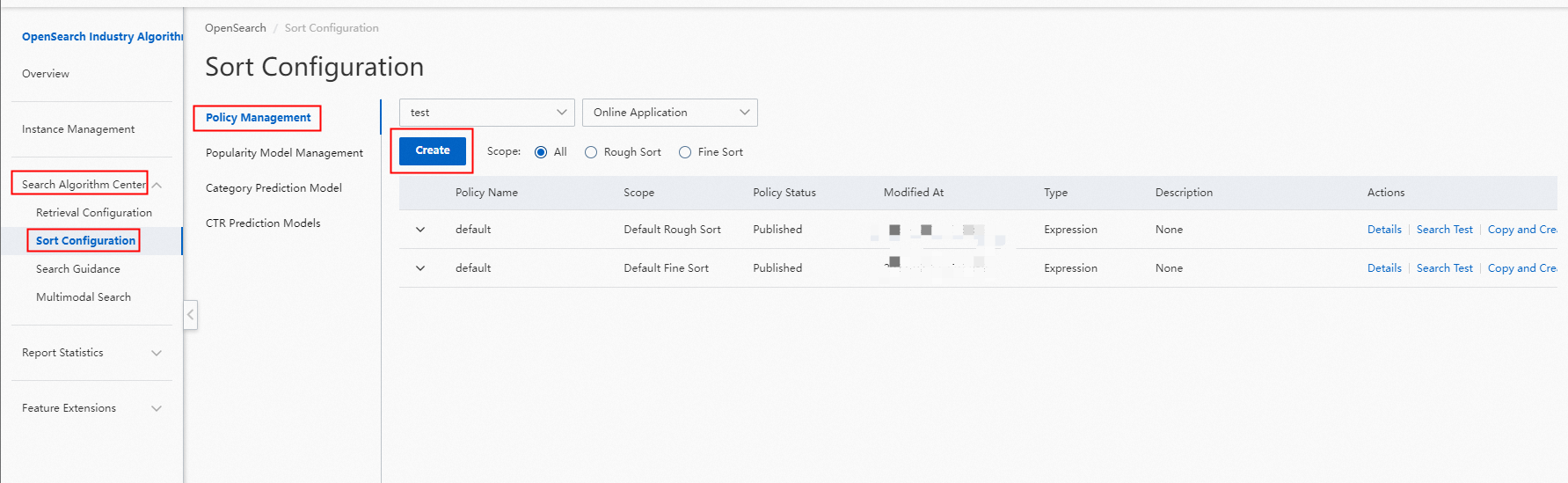In the Sort Configuration step, select static_bm25 from the Scoring Characteristics drop-down list, and set Weight to 10. If the weight is set to 10, the score is multiplied by 10 in the calculation.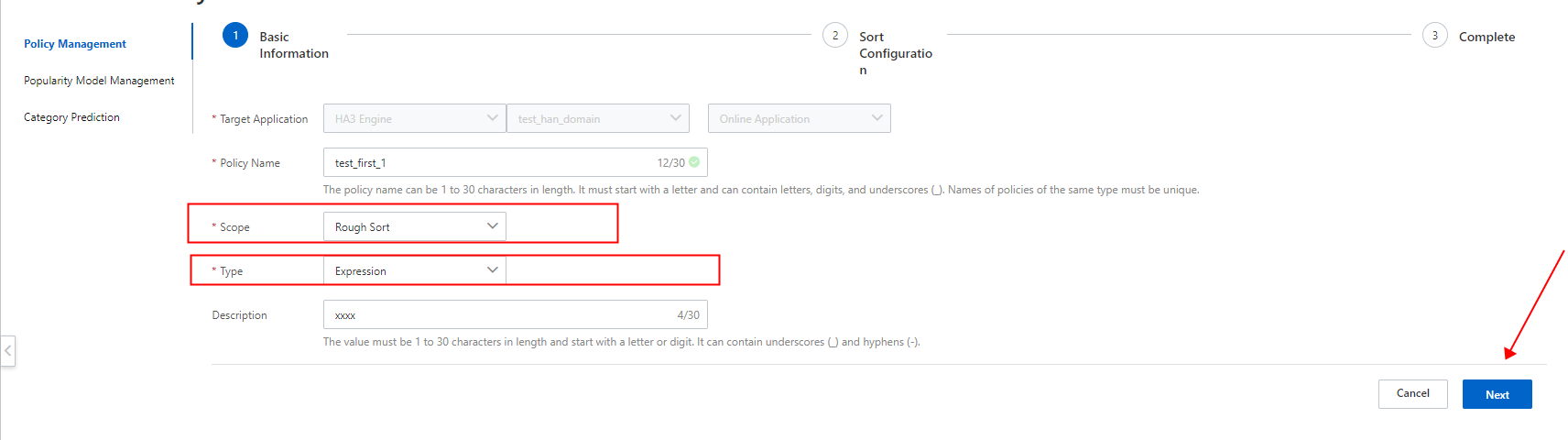You can also specify the search field and set the weight. The specified field must be an attribute field, and only numeric fields are supported, such as fields of the INT, DOUBLE, and FLOAT types. The score of the field value multiplied by the weight is also added to the score.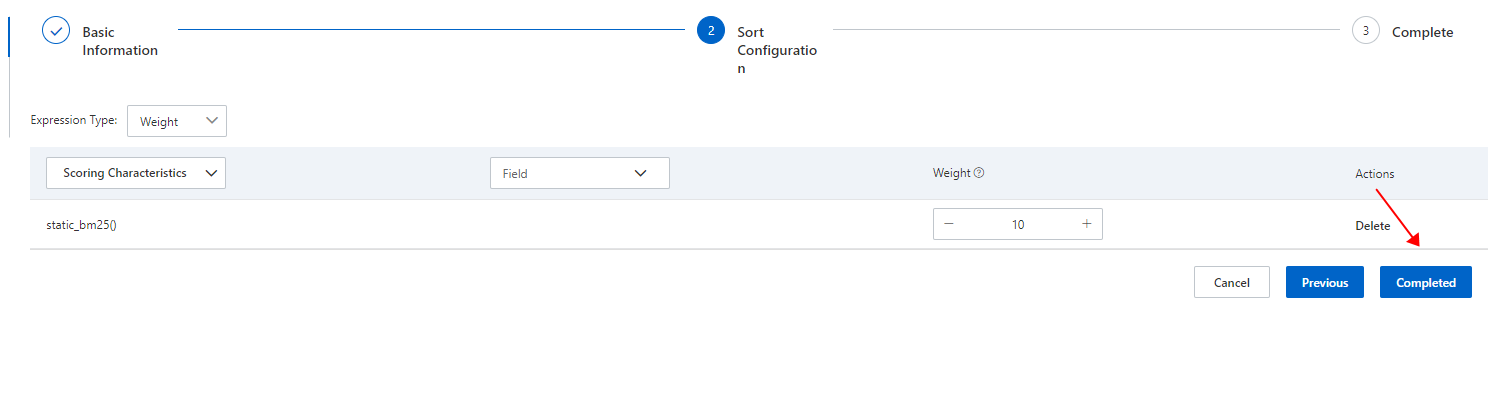After the configuration is complete, click Back to return to the Policy Management page.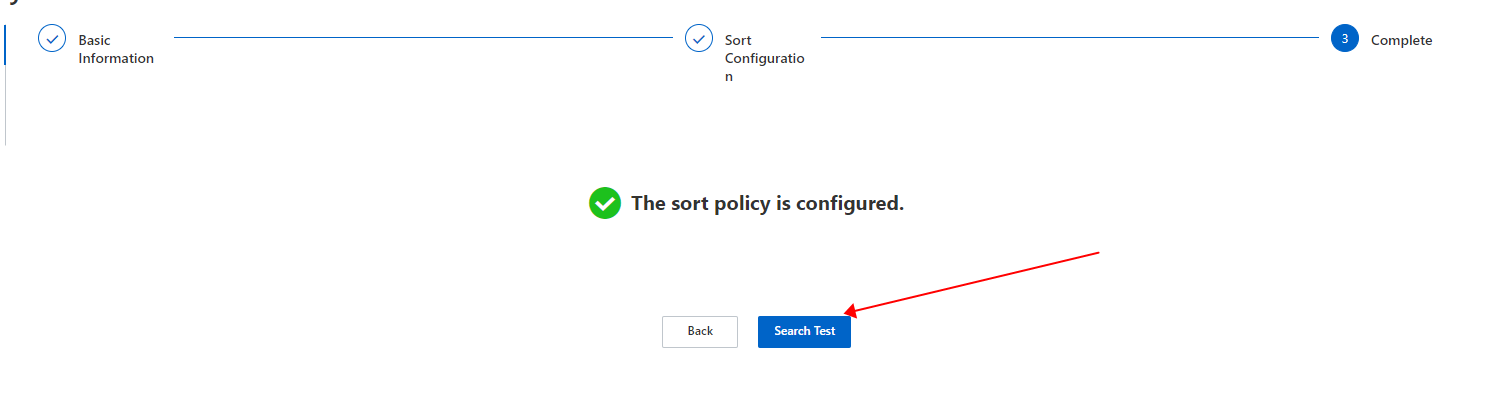2. Create a fine sort policy: Log on to the OpenSearch console. In the left-side navigation pane, choose Search Algorithm Center > Sort Configuration. On the Policy Management page, click Create. In the Basic Information step on the Create Policy page, specify the policy name and set the Scope parameter to Fine Sort and the Type parameter to Expression. Then, click Next.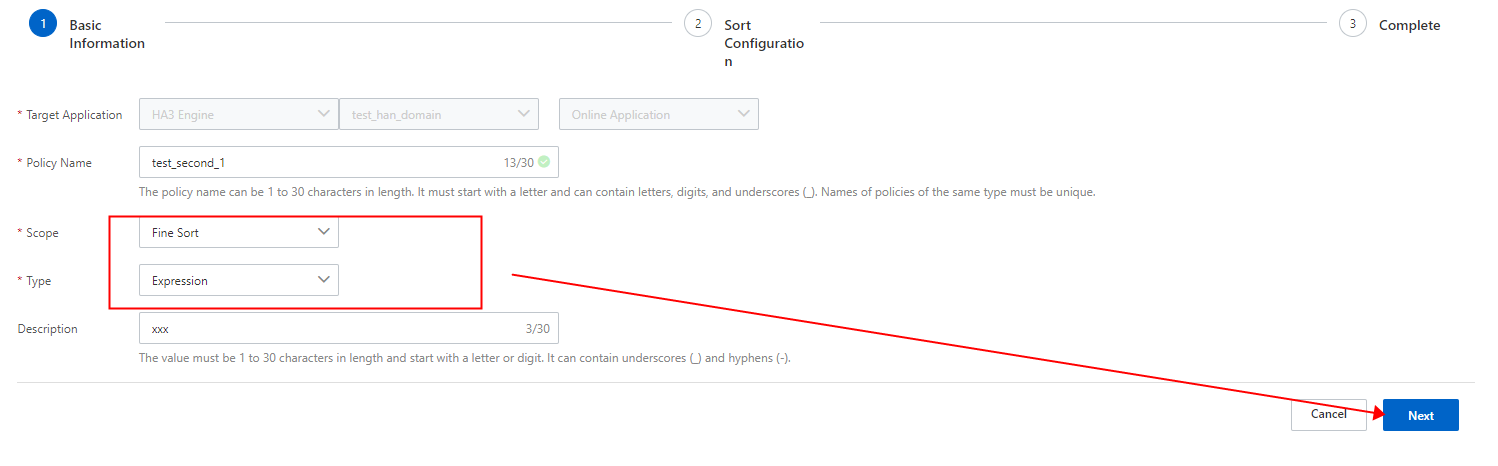In the Sort Configuration step, select text_relevance (field_name) from the Built-in Functions drop-down list, enter the field name to be queried in parentheses, and then click Completed.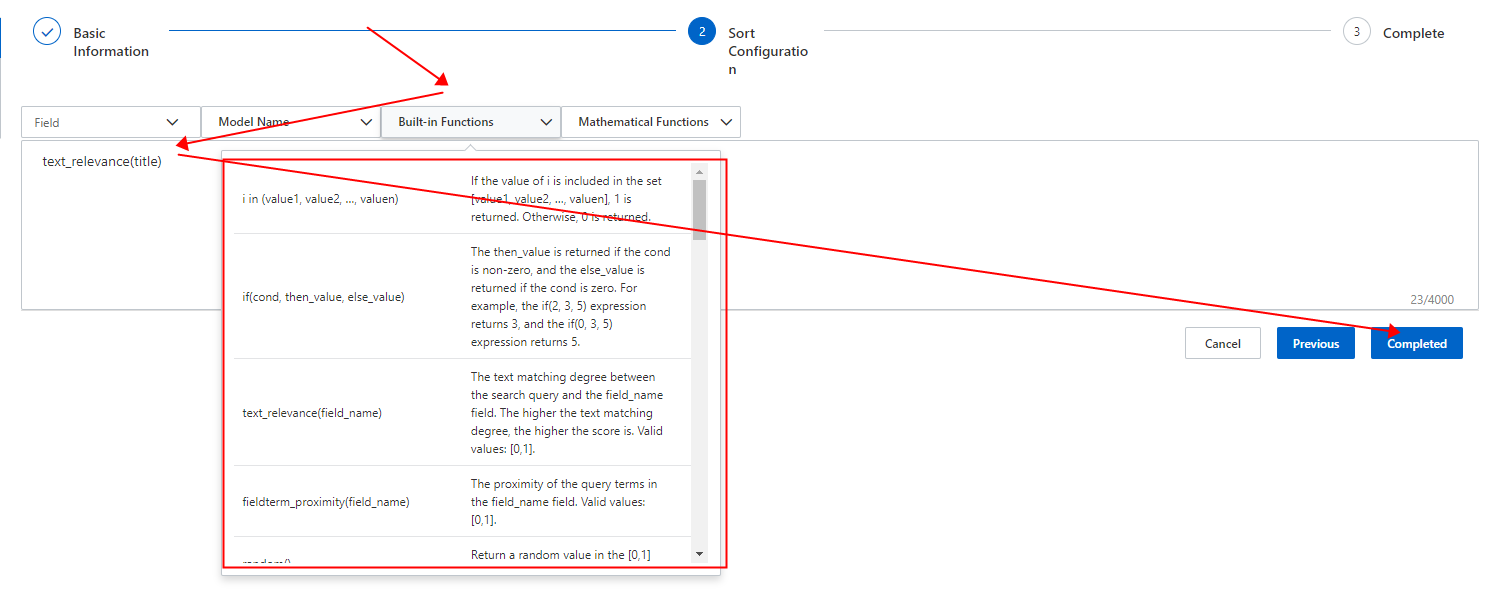After the configuration is complete, click Back to return to the Policy Management page.3. View sort results: On the Search Test page, set the fields for rough sort and fine sort and turn on Show Sort Details.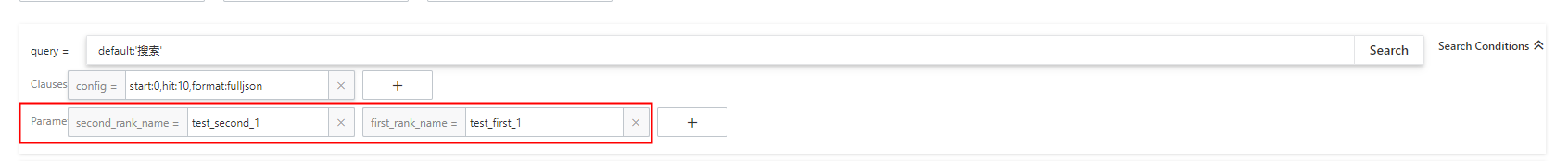The following figure shows the calculated score of each function.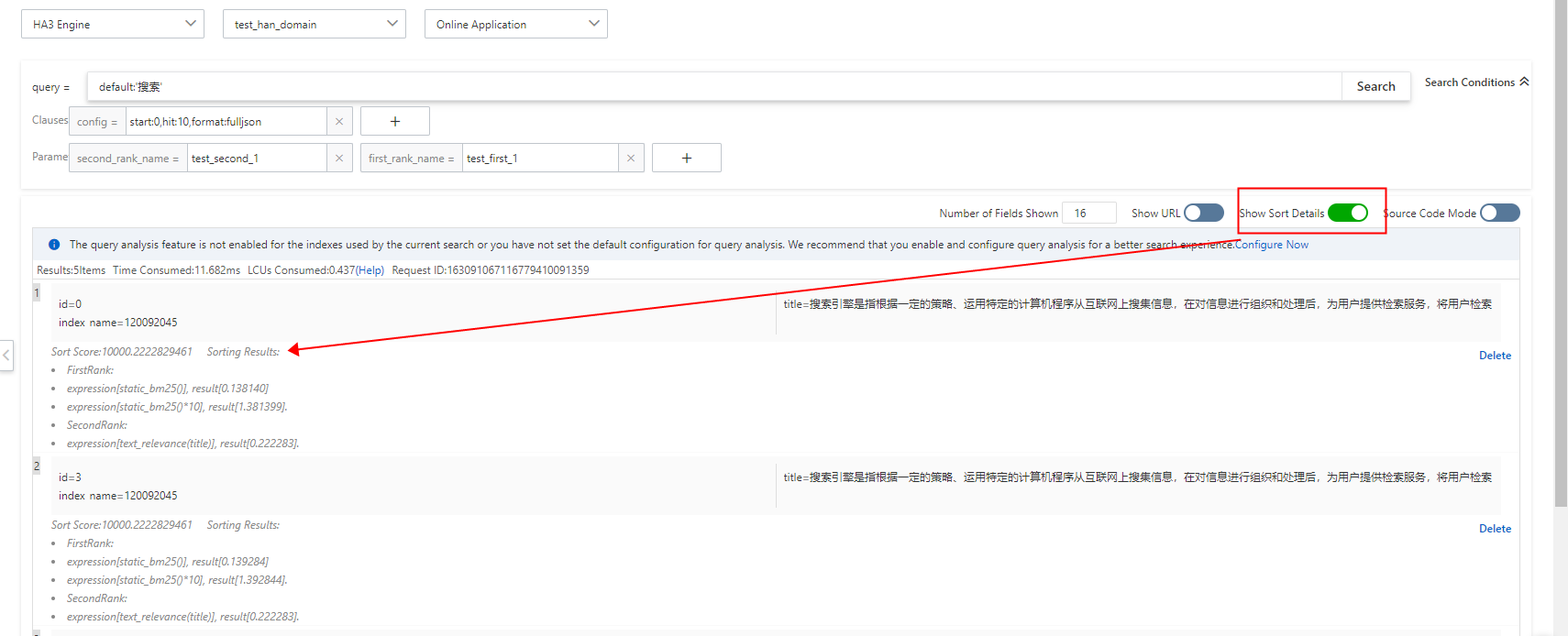Note

Documents are roughly sorted and then finely sorted based on the score. Documents that are retrieved through a query and filtered by a filter enter the rough sort stage. That is, top N high-quality documents are selected from these documents based on the scores calculated by using the rough sort expression. Then, the fine sort expression is used to return the documents that best match the requirements of users. The score is calculated as follows:

• If only a rough sort policy is configured, the document score equals 10,000 plus the result calculated by using the rough sort expression. The maximum document score is 20,000. If the actual document score exceeds 20,000, the displayed score is still 20,000.

• If only a fine sort policy is configured, the document score equals 10,000 plus the result calculated by using the fine sort expression. No upper limit exists for the document score.

• If both a rough sort policy and a fine sort policy are configured, the final score of a document that enters the fine sort stage equals 10,000 plus the result calculated by using the fine sort expression, and the final score of other documents that are only roughly sorted equals 10,000 plus the results calculated by using the rough sort expression. The maximum final score is 20,000. If the actual document score exceeds 20,000, the displayed score is still 20,000.

• You can create multiple rough sort and fine sort rules. However, you can use only one rough sort rule and one fine sort rule at the same time in a query.

Important
• You can specify only one rough sort policy name in first_rank_name. Multiple rough sort expressions cannot be used at the same time.

• You can specify only one fine sort policy name in second_rank_name. Multiple fine sort expressions cannot be used at the same time.

## SDK configurations

SDK for Java:

``````// Specify a rough sort expression and a fine sort expression. In this example, the default expressions are used.
Rank rank =newRank();
rank.setFirstRankName("default");// The name of the rough sort policy
rank.setSecondRankName("default");// The name of the fine sort policy
rank.setReRankSize(5);// Specify the number of documents to be sorted based on the fine sort expression.``````

SDK for PHP:

``````// Specify a rough sort expression.
\$params->setFirstRankName('default');
// Specify a fine sort expression.
\$params->setSecondRankName('default');``````

Usage notes

• The rough and fine sort expressions specified in the code take precedence over the default rough and fine sort expressions configured in the OpenSearch console.

• You can view the sort details of documents by adding a parameter to your code.

Method: Add the format:fulljson parameter to the config clause.

In the return results, the sortExprValues parameter indicates the sort information of a document.The value of the sortExprValues parameter is an array, which is the value of the sort field in the sort clause. Example:

``sort=-price;-RANK``

In this case, the value of the sortExprValues parameter is in the format of [price, document score].

If you do not configure the sort clause, the value of the sortExprValues parameter is the document score by default.